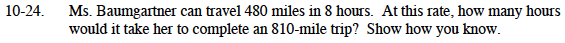### Home > MC1 > Chapter 10 > Lesson 10.1.2 > Problem10-24

10-24.First, you can determine the speed (or the rate).

$\frac{480\text{ miles}}{8\text{ hours}}=60\text{ miles per hour}$

At this speed, how long will it take to travel 810 miles?

$\frac{810}{60}=13.5\text{ hours}$

Another method would be to set up and solve this proportion.

$\frac{480\text{ miles}}{8\text{ miles}}=\frac{810\text{ miles}}{x\text{ hours}}$

Why would this proportion make sense? Try it and see if you get the same answer.

$13\frac{1}{2}\text{ hours}$